• 函数 fLOESS 对一维数据执行 LOESS（使用二阶多项式的局部加权非参数回归拟合）平滑，无需 Matlab 曲线拟合工具箱。 这可能被认为是 LOWESS 的一种稍微更好的方法，它使用线性拟合产生局部加权回归。matlab
• 配套的平滑器 IRLSSMOOTH (ID:49788) 将这种平滑方法更进一步。 用户以样本为单位指定更平滑的响应时间，这将转化为与该多个样本的移动平均值大致相同的带宽。 由于更大的高频衰减，输出更平滑。 或者，用户可以...matlab
• 数据进行平滑处理的典型例子 对数据进行平滑处理的典型例子 对数据进行平滑处理的典型例子
• 2.12 真实数据噪声平滑处理 前言：来自各种真实世界传感器的数据通常是不平滑和不...平滑数据噪声的一个简单朴素的方法就是：对窗口（样本）求平均值；然后绘制出给定窗口的平均值，而不是所有数据点。 代码实现： "
2.12 真实数据的噪声平滑处理
前言：来自各种真实世界传感器的数据通常是不平滑和不干净的，也包含了一些我们不想在显示在图表或者图形的噪声。
1、操作步骤
（1）基础算法是基于滚动窗口模式（rolling window） （2）窗口滚动过数据，然后计算出窗口内数据的平均值 （3）对于离散数据，我们使用Numpy的convolve方法；它返回两个一维序列的离散纤细卷积。
2、工作原理
平滑数据噪声的一个简单朴素的方法就是：对窗口（样本）求平均值；然后绘制出给定窗口的平均值，而不是所有数据点。
代码实现：
"""
真实数据噪声平滑处理
"""
from pylab import *
from numpy import *

def moving_average(interval,window_size):
'''
计算给定大小的卷积窗口
:param interval:
:param window_size:
:return:
'''
#方法ones定义了一个所有元素值为1的序列或者矩阵（例如多维数组）。
# 我们用它来生成用于求平均值的窗口。
window=ones(int(window_size))/float(window_size)
return convolve(interval,window,'same')

#在指定的时间间隔内得到一些均匀间隔的数字。
t=linspace(-4,4,100)
y=sin(t)+randn(len(t))*0.1

plot(t,y,"k.")
#计算移动平均数
y_av=moving_average(y,10)
plot(t,y_av,"r")
xlabel("Time")
ylabel("value")
grid(True)
show()

运行测试：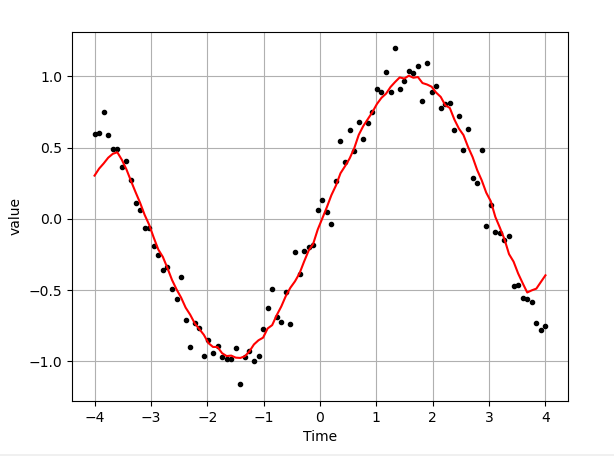从图上我们可以看出平滑数据处理后的曲线和原始数据之间的对比情况。
3、沿着上面的思路，我们可以开始一个更高级的例子 （1）基于信号窗口的卷积
代码实现：
"""
使用现有的SciPy库来让窗口平滑处理达到更好的效果
"""
import numpy
from numpy import *
from pylab import *
#可能的窗口类型
WINDOWS=['flat','hanning','hamming','bartlett','blackman']
def smooth(x,window_len=11,window='hanning'):
"""
使用要求大小的窗口平滑数据。返回平滑信号。
:param x:输入信号
:param window_len:平滑窗长
:param window:窗口类型
:return:
"""
if x.ndim!=1:
raise(ValueError,"smooth只接受一维数组")
if x.size<window_len:
raise(ValueError,"输入向量需要大于窗口大小")
if window_len<3:
return x
if not window in WINDOWS:
raise (ValueError,"Window is one of 'flat', 'hanning', 'hamming', "
"'bartlett', 'blackman'")
#在前面和后面添加反射窗口,减小了数据的边界效应
s=numpy.r_[x[window_len-1:0:-1],x,x[-1:-window_len:-1]]
#选择窗口类型并做平均
if window=='flat':
w=numpy.ones(window_len,'d')
else:
#在numpy中调用适当的函数(call appropriate function in numpy)
w=eval('numpy.'+window+'(window_len)')
#注：长度（输出）=长度（输入），要更正：
#返回y[(window_len/2-1):-(window_len/2)]而不仅仅是y
y=numpy.convolve(w/w.sum(),s,mode='valid')
return y
#在指定的时间间隔内得到一些均匀间隔的数字。
t=linspace(-4,4,100)
#制造一些嘈杂的正弦
x=sin(t)
xn=x+randn(len(t))*0.1
#把它磨平
y=smooth(x)
ws=31
subplot(211)
plot(ones(ws))

#画在相同的轴上(draw on the same axes)
def hold(param):
pass

hold(True)
#绘制每个窗口（plot for every windows）
for w in WINDOWS[1:]:
eval('plot('+w+'(ws) )')
#配置轴属性
axis([0,30,0,1.1])
legend(WINDOWS)
title("Smoothing windows")

subplot(212)
# 绘制原始信号
plot(x)
#绘制加噪信号
plot(xn)
for w in WINDOWS:
plot(smooth(xn,10,w))
#为每个图添加图例
l=['original signal','signal with noise']
l.extend(WINDOWS)
legend(l)

title('Smoothed signal')

show()

运行测试：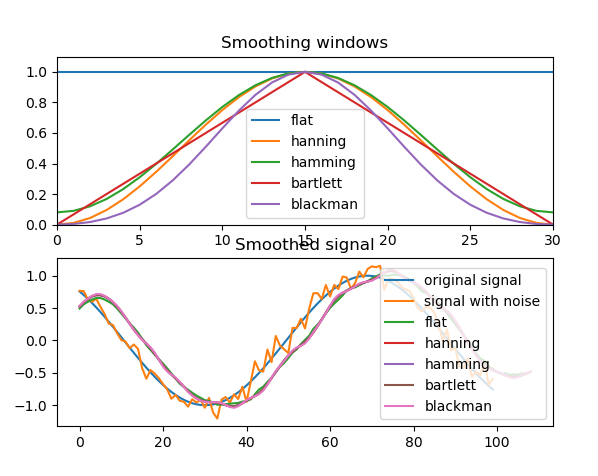可以看出窗口算法是如何影响噪声信号的。上面的图形显示了窗口算法，下面的图形显示了每一个相应的结果，包括原始信号、添加了噪声的信号和经过每个算法平滑处理过的信号
展开全文scipy python numpy
• 当用户移动幻灯片时，P 值会发生变化，并且平滑会根据 P 值和变量上的噪声量而改善或恶化。 在打开滑块值范围从 0 到 1 时，用户可以重置 P 的最小值和最大值。用户可以设置 GUI 以使用对数计算 P 值。 这是通过在...matlab
• ## 数据平滑方法的原理和应用

万次阅读 多人点赞 2019-09-06 15:30:45
文章目录一、简介二、滑动平均法三、指数滑动平均法四、SG滤波法附录 ...滑动平均其实是一个很朴素的方法，但是要与实际结合，构造出合适的平滑方式，是需要一些思考的。下面我将分别介绍滑动平均法（Mean Averag...


文章目录
一、简介二、滑动平均法三、指数滑动平均法四、SG滤波法附录

一、简介
在实际的工程应用中，经常会遇到初始结果噪声太多的问题，比如信号强度抖动的太厉害，比如视频流中的bbox抖动的太厉害，比如光谱信号抖动的太厉害等等，这时候就需要一些简单的滑动平均算法。滑动平均其实是一个很朴素的方法，但是要与实际结合，构造出合适的平滑方式，是需要一些思考的。下面我将分别介绍滑动平均法（Mean Average）、指数滑动平均法（Exponential Mean Average）、SG滤波法（Savitzky Golay Filter）。
二、滑动平均法
简单来说，滑动平均法把前后时刻的一共2n+1个观测值做平均，得到当前时刻的滤波结果。这是一个比较符合直觉的平滑方法，在生活中、工作中很经常会用到，但是很少去思考这么做的依据是什么，下面我就来仔细分析一下其中的原理。 对于一个观测序列，我们有这样的假设：每一次的观测值是带有噪声的，而我们期望噪声的均值为0，方差为

σ

2

\sigma^{2}

，观测值和真实值之间的关系如下：

g

t

=

x

t

+

ε

t

(

1

)

g_{t}=x_{t}+\varepsilon_{t}\space\space\space\space\space\space(1)

其中，

x

t

x_{t}

为观测值，

g

t

g_{t}

为真实值，

ε

t

\varepsilon_{t}

为噪声。为了降低噪声的影响，我们把相邻时刻的观测值相加后平均，公式如下：

p

t

=

∑

i

=

1

n

(

x

t

−

i

+

x

t

+

i

)

+

x

t

2

n

+

1

(

2

)

p_{t}=\frac{\sum_{i=1}^{n}{(x_{t-i}+x_{t+i})+x_{t}}}{2n+1}\space\space\space\space\space\space(2)

p

t

p_{t}

表示 t 时刻的滤波结果，

x

t

−

1

x_{t-1}

表示 t-1 时刻的观测值， n 代表滑动窗口半径。将公式（1）代入公式（2），可以得到

p

t

=

∑

i

=

1

n

(

g

t

−

i

+

g

t

+

i

)

+

g

t

−

∑

i

=

1

n

(

ε

t

−

i

+

ε

t

+

i

)

−

ε

t

2

n

+

1

(

3

)

p_{t}=\frac{\sum_{i=1}^{n}{(g_{t-i}+g_{t+i})}+g_{t}-\sum_{i=1}^{n}{(\varepsilon_{t-i}+\varepsilon_{t+i})}-\varepsilon_{t}}{2n+1}\space\space\space\space\space\space(3)

前面说到了，我们假设噪声的均值为0，所以

∑

i

=

1

n

(

ε

t

−

i

+

ε

t

+

i

)

+

ε

t

\sum_{i=1}^{n}{(\varepsilon_{t-i}+\varepsilon_{t+i})+\varepsilon_{t}}

为0，那么我们得到的结果就是:

p

t

=

∑

i

=

1

n

(

g

t

−

i

+

g

t

+

i

)

+

g

t

2

n

+

1

(

4

)

p_{t}=\frac{\sum_{i=1}^{n}{(g_{t-i}+g_{t+i})}+g_{t}}{2n+1}\space\space\space\space\space\space (4)

当观测数据的真实值变化较小时，或者变化为线性时，可以近似认为：

p

t

=

∑

i

=

1

n

(

g

t

−

i

+

g

t

+

i

)

+

g

t

2

n

+

1

=

g

t

(

5

)

p_{t}=\frac{\sum_{i=1}^{n}{(g_{t-i}+g_{t+i})}+g_{t}}{2n+1}=g_{t}\space\space\space\space\space\space (5)

从上面的分析过程我们可以看到，当滑动窗口内的真实数据变化不大的时候，我们可以抑制掉很大一部分噪声，滤波结果近似真实值；当滑动窗口内的真实值变化较大时，这种滤波方式就会损失一部分精确度，滤波结果接近真实值的平均期望。所以，窗口的大小会对滤波结果有很大影响。窗口越大，滤波结果越平滑，但会一定程度上偏离真实值；窗口越小，滤波结果越接近观测值，但噪声偏大。
滑动平均法还有一个升级版本，也就是加权滑动平均法。实际场景中，每个观测值的重要程度不同，忽略每个观测值的置信度直接平均不能得到精确的结果，所以就需要给观测值加权。加权滑动平均法的公式如下：

p

t

=

∑

i

=

1

n

(

x

t

−

i

∗

w

t

−

i

+

x

t

+

i

∗

w

t

+

i

)

+

x

t

∗

w

t

2

n

+

1

(

6

)

p_{t}=\frac{\sum_{i=1}^{n}{(x_{t-i}*w_{t-i}+x_{t+i}*w_{t+i})+x_{t}*w_{t}}}{2n+1} \space\space\space\space\space\space (6)

w

t

w_{t}

为 t 时刻的权重。（6）式表示的是把每个观测值乘以权重后再平均。这种方法适用于观测值本身带有置信度的情况。注意，这里有一个小问题，如果置信度的取值范围是0到1之间，那么加权之后计算得到的观测值往往小于真实值，我来解释一下为什么。首先，我们假设观测值和真实值的均值是相等的，也就是

X

ˉ

=

∑

i

=

1

2

n

+

1

x

t

2

n

+

1

=

∑

i

=

1

2

n

+

1

g

t

2

n

+

1

=

G

ˉ

(

7

)

\bar{X} = \frac{\sum_{i=1}^{2n+1}{x_{t}}}{2n+1}=\frac{\sum_{i=1}^{2n+1}{g_{t}}}{2n+1} = \bar{G} \space\space\space\space\space\space (7)

当我们把观测值乘以权重了之后，观测值和真实值的均值就不相等了，因为真实值的权重均值为1，而观测值的权重均值为

w

t

ˉ

=

∑

i

=

1

n

(

w

t

−

i

+

w

t

+

i

)

+

w

t

2

n

+

1

\bar{w_{t}}=\sum_{i=1}^{n}\frac{(w_{t-i}+w_{t+i})+w_{t}}{2n+1}

，是小于等于1的，最终的预测值也是小于等于真实值的，而且大概率是小于。所以我们需要对（6）式增加一个修正：

p

t

~

=

p

t

w

t

ˉ

(

8

)

\tilde{p_{t}} = \frac{p_{t}}{ \bar{ w_{t}} } \space\space\space\space\space\space (8)

这样，得到的预测值就会更加合理了。
小结：滑动平均法使用的前提是，噪声的均值为0，真实值变化不大或线性变化的场景。如果真实值有较高频率的非线性突变的话，滑动平均法的效果就不够好了。同时，滑动平均法的窗口选取很重要，需要根据具体数据来选择。如果需要使用在线版本的滑动平均，那么就要把窗口前移，也就是把当前时刻的前n个观测值进行平均，但这样得到的结果会明显滞后于当前观测值，窗口越大，滞后的现象越严重。
class MovAvg(object):
def __init__(self, window_size=7):
self.window_size = window_size
self.data_queue = []

def update(self, data):
if len(self.data_queue) == self.window_size:
del self.data_queue
self.data_queue.append(data)
return sum(self.data_queue)/len(self.data_queue)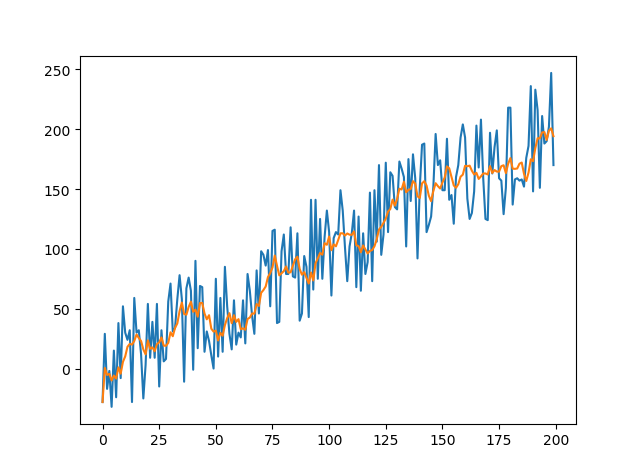三、指数滑动平均法
指数滑动平均法相当于加权滑动平均法的变体，主要区别在于，指数滑动平均法的权重是固定的、随时间推移呈指数衰减。指数滑动平均法的公式如下：

p

t

=

w

∗

x

t

+

(

1

−

w

)

∗

p

t

−

1

(

9

)

p_{t}=w*x_{t}+(1-w)*p_{t-1} \space\space\space\space\space\space (9)

p

t

p_{t}

表示预测值，

w

w

表示衰减权重，通常我们设为固定值0.9，

x

t

x_{t}

表示观测值，这是一个递推公式。前面说了，指数滑动平均法的权重是随时间推移呈指数衰减的，那么上面的这个递推公式的指数体现在哪里呢？我们把（9）式进行延伸：

p

t

−

1

=

w

∗

x

t

−

1

+

(

1

−

w

)

∗

p

t

−

2

(

10

)

p_{t-1}=w*x_{t-1}+(1-w)*p_{t-2} \space\space\space\space\space\space (10)

将（9）和（10）两式子联立，可得

p

t

=

w

∗

x

t

+

(

1

−

w

)

∗

(

w

∗

x

t

−

1

+

(

1

−

w

)

∗

p

t

−

2

)

(

11

)

p_{t}=w*x_{t}+(1-w)*(w*x_{t-1}+(1-w)*p_{t-2}) \space\space\space\space\space\space (11)

发现没有，在（11）式中

p

t

p_{t}

与

p

t

−

2

p_{t-2}

的关系是

(

1

−

w

)

2

(1-w)^{2}

倍，而在（9）式中

p

t

p_{t}

与

p

t

−

1

p_{t-1}

的关系是

1

−

w

1-w

倍，呈指数衰减关系。同时，在初始时刻有如下关系：

p

0

=

x

0

(

12

)

p_{0}=x_{0} \space\space\space\space\space\space (12)

根据这一关系和上述的递推公式，我们就能够得到整个算法的公式了。
由于这种指数衰减的特性，指数滑动平均法会比滑动平均法的实时性更强，更加接近当前时刻的观测值。在实际场景下，如果目标的波动较大时，指数滑动平均法会比滑动平均更加接近当前的真实值。那么是不是就说明，指数滑动平均法在任意场景下都比滑动平均法更好呢？不一定。我们来分析一下指数衰减法的误差项，这里为了简便表示，设定 t=2 ，同时，将（1）式和（12）式代入公式（11），可得到误差项：

ε

=

w

∗

ε

2

+

(

1

−

w

)

∗

(

w

∗

ε

1

+

(

1

−

w

)

∗

ε

0

)

(

13

)

\varepsilon=w*\varepsilon_{2}+(1-w)*(w*\varepsilon_{1}+(1-w)*\varepsilon_{0}) \space\space\space\space\space\space (13)

所以误差项也是呈指数衰减的，越接近当前时刻的误差项权重越大。假如在当前的工程场景中，误差是固定的分布，不受目标的观测值大小影响的话，那么指数滑动平均法会更接近真实值；假如误差会受目标观测值影响，比如我们观测的是一个连续运动的目标，中间突然出现了一个偏离很远的观测点，那么这个点为误检的概率相当大，也就是该观测值的误差比之前其他点的误差要大得多，此时指数加权平均法的结果就会波动较大，结果就不如滑动平均了。
小结：当误差不受观测值大小影响的话，指数滑动平均比滑动平均好；当误差随观测值大小变化时，滑动平均比指数滑动平均更好。
class ExpMovAvg(object):
def __init__(self, decay=0.9):
self.decay = decay
self.first_time = True

def update(self, data):
if self.first_time:
self.first_time = False
return data
else: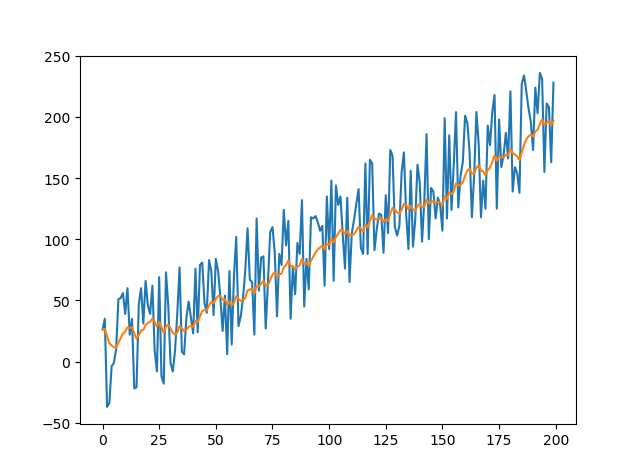四、SG滤波法
SG滤波法（Savitzky Golay Filter）的核心思想也是对窗口内的数据进行加权滤波，但是它的加权权重是对给定的高阶多项式进行最小二乘拟合得到。它的优点在于，在滤波平滑的同时，能够更有效地保留信号的变化信息，下面我来介绍一下其原理。 我们同样对当前时刻的前后一共2n+1个观测值进行滤波，用k-1阶多项式对其进行拟合。对于当前时刻的观测值，我们用下面的公式进行拟合：

x

t

=

a

0

+

a

1

∗

t

+

a

2

∗

t

2

+

.

.

.

+

a

k

−

1

∗

t

k

−

1

(

14

)

x_{t}=a_{0}+a_{1}*t+a_{2}*t^{2}+...+a_{k-1}*t^{k-1} \space\space\space\space\space\space (14)

同样，对于前后时刻（如t-1、t+1、t-2、t+2等时刻）的预测值，我们同样可以用（14）式来计算，这样一共得到2n+1个式子，构成一个矩阵（似乎发不了矩阵，我放个图片吧）：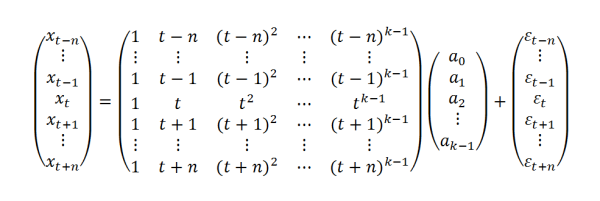要使得整个矩阵有解，必须满足 2n+1>k，这样我们才能够通过最小二乘法确定参数

a

0

a_{0}

、

a

1

a_{1}

、

a

2

a_{2}

、…

a

k

−

1

a_{k-1}

。我们把上面的矩阵简化表示为下面公式：

X

(

2

n

+

1

)

×

1

=

T

(

2

n

+

1

)

×

k

+

A

k

×

1

+

E

(

2

n

+

1

)

×

1

(

15

)

X_{(2n+1)\times1}=T_{(2n+1)\times k}+A_{k\times1}+E_{(2n+1)\times1} \space\space\space\space\space\space (15)

各个参数下标表示它们各自的维度，如

A

k

×

1

A_{k\times1}

表示有k行1列的参数。通过最小二乘法，我们可以求得

A

k

×

1

A_{k\times1}

的解为：

A

=

(

T

t

r

a

n

s

⋅

T

)

−

1

⋅

T

t

r

a

n

s

⋅

X

(

16

)

A=(T^{trans}\cdot T)^{-1} \cdot T^{trans} \cdot X \space\space\space\space\space\space (16)

上标

t

r

a

n

s

trans

表示转置。那么，模型的滤波值为：

P

=

T

⋅

A

=

T

⋅

(

T

t

r

a

n

s

⋅

T

)

−

1

⋅

T

t

r

a

n

s

⋅

X

=

B

⋅

X

(

17

)

P=T\cdot A=T\cdot (T^{trans}\cdot T)^{-1} \cdot T^{trans}\cdot X =B\cdot X \space\space\space\space\space\space (17)

最终可以得到滤波值和观测值之间的关系矩阵：

B

=

T

⋅

(

T

t

r

a

n

s

⋅

T

)

−

1

⋅

T

t

r

a

n

s

(

18

)

B=T\cdot (T^{trans}\cdot T)^{-1} \cdot T^{trans} (18)

算出了B矩阵，我们就能够快速的将观测值转换为滤波值了。 小结：SG滤波法对于数据的观测信息保持的更好，在一些注重数据变化的场合会比较适用。
class SavGol(object):
def __init__(self, window_size=11, rank=2):
assert window_size % 2 == 1
self.window_size = window_size
self.rank = rank

self.size = int((self.window_size - 1) / 2)
self.mm = self.create_matrix(self.size)
self.data_seq = []

def create_matrix(self, size):
line_seq = np.linspace(-size, size, 2*size+1)
rank_seqs = [line_seq**j for j in range(self.rank)]
rank_seqs = np.mat(rank_seqs)
kernel = (rank_seqs.T * (rank_seqs * rank_seqs.T).I) * rank_seqs
mm = kernel[self.size].T
return mm

def update(self, data):
self.data_seq.append(data)
if len(self.data_seq) > self.window_size:
del self.data_seq
for i in range(left):
for i in range(right):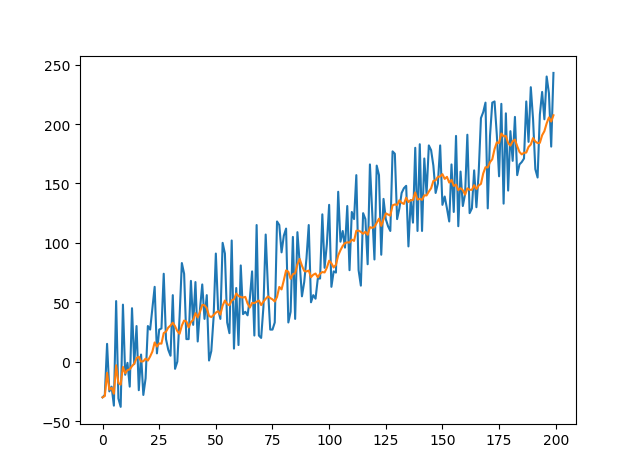附录
本文图片制作的相关代码。
import cv2
import numpy as np
import matplotlib.pyplot as plt

import imageio

# 一维数据滤波
ma, ema, sg = MovAvg(), ExpMovAvg(), SavGol()
data_list, data_ma, data_ema, data_sg = [], [], [], []
for i in range(200):
data = i+np.random.randint(-50, 50)
data_list.append(data)
data_ma.append(ma.update(data))
data_ema.append(ema.update(data))
data_sg.append(sg.update(data))
plt.plot(data_list, label='raw')
plt.plot(data_ma, label='ma')
plt.plot(data_ema, label='ema')
plt.plot(data_sg, label='sg')
plt.legend()
plt.show()

# 鼠标轨迹滤波
ma_x, ma_y = MovAvg(), MovAvg()
ema_x, ema_y = ExpMovAvg(), ExpMovAvg()
sg_x, sg_y = SavGol(), SavGol()

def draw_circle(event, x, y, flags, param):
if event == cv2.EVENT_MOUSEMOVE:
sx = np.random.randint(-50, 51)
sy = np.random.randint(-50, 51)
cv2.circle(show, (x+sx, y+sy), 5, (255, 255, 255), -1)
x, y = ma_x.update(x+sx), ma_y.update(y+sy)
cv2.circle(show, (int(x), int(y)), 5, (0, 0, 255), -1)
x, y = ema_x.update(x+sx), ema_y.update(y+sy)
cv2.circle(show, (int(x), int(y)), 5, (0, 255, 0), -1)
x, y = sg_x.update(x+sx), sg_y.update(y+sy)
cv2.circle(show, (int(x), int(y)), 5, (255, 0, 0), -1)

show = np.zeros((1024, 1024, 3), np.uint8)
cv2.namedWindow('image')
buff = []
while True:
cv2.setMouseCallback('image', draw_circle)
cv2.imshow('image', show)
save = cv2.resize(show, (512, 512))
save = cv2.cvtColor(save, cv2.COLOR_BGR2RGB)
buff.append(save)
if cv2.waitKey(100) == ord('q'):
break
cv2.destroyAllWindows()
imageio.mimwrite('test.gif', buff, 'GIF', duration=0.1)


展开全文滤波算法 指数滑动平均 SG滤波
• 在做数据分析时, 由于数据噪声太多, 需要对数据进行数据平滑处理. 通常包含有降噪/拟合等操作. 降噪的功能在于去除额外的影响因素. 拟合的目的在于数学模型化,可以通过更多的数学方法识别曲线特征. 降噪手段: 卷积...
数据分析-数据平滑处理
数据平滑处理
在做数据分析时, 由于数据的噪声太多, 需要对数据进行数据平滑处理. 通常包含有降噪/拟合等操作. 降噪的功能在于去除额外的影响因素. 拟合的目的在于数学模型化,可以通过更多的数学方法识别曲线特征.
降噪手段: 卷积运算
# 通常卷积核的选取API
con_core = np.hanning(8)
con_core /= con_core.sum()
# 卷积API
np.convolve(samples, con_core, 'valid')

数学模型化: 多项式拟合
# 通过原函数曲线(x,y)/最高次数 得到 拟合多项式系数
P = np.polyfit(x, y, n)
# 通过多项式系数与x的值,求对应的多项式函数值.
y = np.polyval(P, x)
# 通过原函数求导函数
Q = np.polyder(P)
# 求多项式的根
X = np.roots(Q)
# 求两个多项式函数的差函数. 可以通过该方法求取两个多项式
# 函数的交点
Q = np.polysub(P1, P2)

符号数组
sign函数可以把样本数组变成对应的符号数组, 所有正数变为1, 负数变为-1, 0还是0.
ary = np.sign(源数组)

绘制 净额成交量(OBV)
若相比上一天的收盘价上涨,则为正成交量; 若比上一天的收盘价下跌,则为负成交量.
成交量可以反映市场对某支股票的人气, 成交量是一支股票上涨的能量. 一般情况下股票上涨往往需要较大的成交量,而下跌时则不然.
案例: 绘制OBV柱状图
import numpy as np
import matplotlib.pyplot as mp
import datetime as dt
import matplotlib.dates as md

def dmy2ymd(dmy):
dmy = str(dmy, encoding='utf-8')
date = dt.datetime.strptime(
dmy, '%d-%m-%Y').date()
s = date.strftime("%Y-%m-%d")
return s

# 加载文件
'../data/bhp.csv', delimiter=',',
usecols=(1, 6, 7), unpack=True,
dtype='M8[D], f8, f8',
converters={1: dmy2ymd})

# 获取相比上一天股价是否上涨
diff_closing_prices = np.diff(closing_prices)
# 获取相对应的符号数组
sign_closing_prices = np.sign(diff_closing_prices)
# 绘制每天的成交量
obvs = volumes[1:] * sign_closing_prices

# 绘制净额成交量柱状图
mp.figure('OBV', facecolor='lightgray')
mp.title('OBV', fontsize=18)
mp.xlabel('Dates', fontsize=14)
mp.ylabel('Volumes', fontsize=14)
# 整理x轴刻度定位器
ax = mp.gca()
ax.xaxis.set_major_locator(
md.WeekdayLocator(byweekday=md.MO))
ax.xaxis.set_major_formatter(
md.DateFormatter('%d %b %Y'))
ax.xaxis.set_minor_locator(md.DayLocator())

mp.tick_params(labelsize=10)
mp.grid(linestyle=':', axis='y')
dates = dates[1:].astype(md.datetime.datetime)

mp.bar(dates, obvs, 1.0, color='dodgerblue',
edgecolor='white', label='OBV')

mp.gcf().autofmt_xdate()
mp.legend()
mp.show()

数组预处理函数
# array 源数组
# 条件序列:  [a<60, a==60, a>60]
# 取值序列:  [-1,   0,     1   ]
np.piecewise(array, 条件序列, 取值序列)

测试:
a = np.array([23, 94, 65, 23, 84, 56, 23])
d = np.piecewise(
a,
[(20 < a) & (a < 60), a == 60, a > 60],
[-1, 0, 1])
print(d)

矢量化
矢量化指的是用数组代理标量来操作数组里的每个元素.
numpy提供了vectorize函数, 可以把处理标量的函数矢量化. 经过vectorize函数矢量化处理过后将会返回一个矢量函数, 该函数可以直接处理ndarray数组.
案例:
import numpy as np
import math as m

def foo(x, y):
return m.sqrt(x**2 + y**2)

x, y = 3, 4
print(foo(x, y))
x = np.array([3, 4, 5, 6])
y = np.array([4, 5, 6, 7])
# z = foo(x, y)  错误
# 把foo函数矢量化处理
foo_v = np.vectorize(foo)
print(foo_v(x, y))

numpy提供了frompyfunc函数, 也可以完成vectorize相同的功能
# 使用frompyfunc方法矢量化函数
# foo需要2个参数, 最终将会有1个返回值
foo_f = np.frompyfunc(foo, 2, 1)
print(foo_f(x, y))

矩阵
numpy.matrix类型用来描述矩阵. 该类继承自numpy.ndarray. 任何多维数组的操作,对矩阵同样有效. 但是作为子类矩阵又结合了自身的特点,做了必要的扩充,比如乘法计算/矩阵求逆等等.
矩阵的创建
# ary: 任何可以被解释为矩阵的二维容器
# copy: 如果copy的值为True(缺省), 所得到的矩阵对象与参
# 数中源容器各自拥有独立的数据拷贝.否则,共享同一份数据
numpy.matrix(ary, copy=True)

# ary: 任何可以被解释为矩阵的二维容器
# 默认copy=False
numpy.mat(ary)

# str: 字符串矩阵拼块规则
#      '1 2 3;4 5 6;7 8 9'
np.mat(str)

矩阵的乘法运算
'''
a * b
矩阵的乘法:a矩阵的每一行分别乘以b矩阵的每一列
结果矩阵的行数与a的行数一致, 结果矩阵的列数与b的列数一致
'''
e = np.mat('1 2 6; 3 5 7; 4 8 9')
print(e * e)

矩阵的逆矩阵
若两个矩阵A/B满足: AB = BA = E (单位矩阵),则 A/B互为逆矩阵.
单位矩阵: 矩阵的主对角线值为1, 其他位置值为0的矩阵.
e = np.mat(..)
print(e.I)  #逆矩阵

案例 : 春游, 去程做大巴, 小孩票价3元, 家长票价3.2元, 一共花了118.4; 回程做火车, 小孩3.5, 家长3.6, 花了135.2; 求小孩与家长的人数.
\left[ \begin{array}{ccc} x & y \end{array} \right] \times \left[ \begin{array}{ccc} 3 & 3.5 \ 3.2 & 3.6 \ \end{array} \right]
\left[ \begin{array}{ccc} 118.4 & 135.2 \end{array} \right]
斐波那契数列
1 1 2 3 5 8 13 ...

1 1  1 1  1 1  1 1
X  1 0  1 0  1 0  1 0
---------------------------------------------
1 1  2 1  3 2  5 3
1 0  1 1  2 1  3 2  ...

numpy通用函数
加法通用函数
np.add(a, b)			# 两数组相加

案例:
a = np.arange(1, 7)
print(a)

除法通用函数
# 真除
a / b
np.true_divide(a, b)
np.divide(a, b)
# 地板除
np.floor_divide(a, b)
np.ceil(a / b)	# 天花板除
np.trunc(a / b) # 截断除

import numpy as np

a = np.array([20, 20, -20, -20])
b = np.array([3, -3, 6, -6])
print(a)
print(b)
# 开始测试
print(np.true_divide(a, b))
print(np.divide(a, b))

print(np.floor_divide(a, b))
print(np.ceil(a / b))
print(np.trunc(a / b))

三角函数通用函数
np.sin()  np.cos()

傅里叶定理
傅里叶说过, 任何周期函数都可以由多个不同振幅/频率/相位的正弦函数叠加而成.
合成方波
一个方波由如下参数的正弦波叠加而成:
y = 4\pi \times sin(x) \ y = \frac{4}{3}\pi \times sin(3x) \ y = \frac{4}{5}\pi \times sin(5x) \ y = \frac{4}{7}\pi \times sin(7x) \ … \ y = \frac{4}{2n-1}\pi \times sin((2n-1)x) \
案例:
import numpy as np
import matplotlib.pyplot as mp

x = np.linspace(-2 * np.pi, 2 * np.pi, 1000)
# 根据公式搞出来3条曲线
y1 = 4 * np.pi * np.sin(x)
y2 = 4 / 3 * np.pi * np.sin(3 * x)
y3 = 4 / 5 * np.pi * np.sin(5 * x)

# 使用循环控制叠加波的数量
n = 1000
y = np.zeros(1000)
for i in range(1, n + 1):
y += 4 / (2 * i - 1) * np.pi * \
np.sin((2 * i - 1) * x)

mp.figure('SIN', facecolor='lightgray')
mp.title('SIN', fontsize=18)
mp.xlabel('X', fontsize=14)
mp.ylabel('Y', fontsize=14)
mp.tick_params(labelsize=10)

mp.plot(x, y1, label='y1')
mp.plot(x, y2, label='y2')
mp.plot(x, y3, label='y3')
mp.plot(x, y, label='y')

mp.legend()
mp.show()

位运算通用函数
位异或
c = a ^ b
c = np.bitwise_xor(a, b)

按位异或可以很方便的判断两个数据是否同号
a = np.array([4, -6, 7, -3, -4, 2])
b = np.array([-2, -8, 2, -5, 3, -4])

print(a)
print(b)
print(a ^ b)
print(np.bitwise_xor(a, b))
# where找到符合条件的元素下标 (异号)
print(np.where((a ^ b) < 0))

位与
e = a & b
e = np.bitwise_and(a, b)

利用位与运算计算某个数字是否是2的幂
'''
1  2^0  00001    0  00000
2  2^1  00010    1  00001
4  2^2  00100    3  00011
8  2^3  01000    7  00111
'''
print('-' * 40)
d = np.arange(1, 20)
print(d)
e = np.bitwise_and(d, d - 1)
print(e)

位或 / 位反 / 移位
# 位或操作
np.bitwise_or(a, b)
# 位反操作 (1变0, 0变1)
np.bitwise_not(a)
# 移位操作
np.left_shift(array, 1)		#每个元素左移1位 (乘2)
np.right_shift(array, 1)	#每个元素右移1位 (除2)

numpy提供的线性代数模块(linalg)
逆矩阵和广义逆矩阵
如果一个方阵A与方阵B的乘积是单位矩阵,则AB互为逆矩阵.
np.linalg.inv(A)  # 返回A方阵的逆矩阵

如果A不是方阵, A的逆矩阵则称为广义逆矩阵.
np.linalg.pinv(A) # 返回矩阵A的广义逆矩阵

d = A.I # 既可以返回逆矩阵也可以返回广义逆矩阵

线性方程求解与线性拟合
# 求解线性方程组
c = np.linalg.solve(a, b)
# 线性拟合(求出误差最小的结果矩阵)
c = np.linalg.lstsq(a, b)

\begin{cases} x -2y+z=0\ 2y-8z=8\ -4x+5y+9z=-9 \end{cases}
展开全文• 因此，需要对有噪声的原始数据进行数据平滑预处理以消除噪声的影响。 （1）生成一组含噪声的一维时间序列模拟数据：时间 ，时间间隔0.05，时间序列，随机产生一组服从标准正态分布的噪声 。那么，含噪声的时间序列 ...
噪声的平滑处理 在实测数据记录中经常会存在有很多噪声，会导致数据出现突然的抖动等情况。因此，需要对有噪声的原始数据进行数据平滑预处理以消除噪声的影响。 （1）生成一组含噪声的一维时间序列模拟数据：时间 ，时间间隔0.05，时间序列，随机产生一组服从标准正态分布的噪声 。那么，含噪声的时间序列 。 （2）采用要求的平滑预处理算法对 进行降噪处理，得到平滑时间序列 。计算 与 的均方根误差 ，衡量算法的平滑性能，误差越小，平滑性能越好。其中 表示一维数据 的长度， 和 分别表示一维数据 和的第i个数据。 要求：使用移动平均平滑预处理算法，假设输入为x，输出为y，则平滑公式 ，当滑动窗口长度N=4时 ，初始化 、、。 （3）画出两个对比图： 图1画出时间序列与噪声序列的对比曲线图。（ 用蓝色实线； X用绿色实线，菱形标记，标记大小为4，菱形边缘绿色，填充黄色；线宽1.5磅；添加坐标轴名，图名；画出分格线）。 图2画出时间序列与平滑序列的对比曲线图。（ 用蓝色实线；Y用紫色实线，五角星标记，标记大小为4，五角星边缘紫色，线宽1.5磅；添加坐标轴名，图名；画出分格线）。
（知识点：采集数据中的噪声、数据抖动、降噪方法、移动平均平滑算法、均方根误差） 想问下各位大佬们，这个题怎么破？
展开全文• 来自真实世界的传感数据通常是不平滑不干净的，包含一些我们不想显示的噪声 https://blog.csdn.net/bitcarmanlee/article/details/54729807 参考文章 https://blog.csdn.net/shu15121856/article/details/76285479...
• ## 数据处理时如何解决噪声数据？

万次阅读 多人点赞 2019-04-30 10:08:05
在机器学习中我们在独立随机抽样的时候会出现一些搞错的信息，这些错误的数据我们称之为杂讯（或者噪音 noise），一般可以归结为一下两种（以二分为例）： 输出错误： 1.同样的一笔数据会出现两种不同的评判 2....机器学习
• 数据挖掘：数据预处理——数据噪声处理 一、什么是数据噪声数据噪声(Noise)：数据集中的干扰数据（对场景描述不准确的数据），即测量变量中的随机误差或方差。 二、噪声数据与离群点的区别 观测量(Measurement) ...python
• 平滑数据噪声的一个简单朴素的做法是，对窗口（样本）求平均，然后仅仅绘制出给定窗口的平均值，而不是所有的数据点。 import matplotlib.pyplot as plt import numpy as np def moving_average(interval, ...
• ## 几种平滑处理方法

万次阅读 2017-12-07 15:40:53
另一类是消除噪音。空间域的平滑滤波一般采用简单平均法进行，就是求邻近像元点的平均亮度值。邻域的大小与平滑的效果直接相关，邻域越大平滑的效果越好，但邻域过大，平滑会使边缘信息损失的越大，从而使输出的图像...图像处理
• 函数 binAveraging 通过平滑高频范围，可以更清晰地可视化湍流速度密度的功率谱密度估计。 它还可以用于将数据平均到不重叠的 bin 中。 本呈件包含： - 函数 binAveraging.m - 示例文件 Example.mlx - 包含模拟...matlab
• 数据平滑通常对噪声这一块进行处理，平滑的本质就是用来解决零概率问题，尽量使概率分布趋于均匀，使数据变得更可用。 下面介绍几种自己总结的数据平滑方法： 1.分箱法平滑 首先进行数据排序，将他们分到等宽的...机器学习
• 光谱数据预处理，对光谱数据噪声进行平滑操作。去除其中的噪声等，消除干扰因素。得到可以利用的数据预处理
• 在对时间序列数据（如信号数据或股票价格数据）进行统计分析时，往往需要对数据进行平滑处理，本次主要介绍smooth函数、smoothts函数和medfilt1函数的用法 1.smooth函数 smooth函数调用格式如下： 1) yy=smooth(y) ...MATLAB
• python 数据、曲线平滑处理——方法总结Savitzky-Golay 滤波器实现曲线平滑插值法对折线进行平滑曲线处理基于Numpy.convolve实现滑动平均滤波数据平滑处理——log()和exp()函数 问题描述： 在寻找曲线的波峰、波谷时...
• ## MATLAB中数据平滑处理

万次阅读 多人点赞 2017-05-05 14:31:40
smoothts函数 调用格式： output = smoothts(input) output = smoothts(input, ‘b’, ...output = smoothts(input, ‘g’, wsize, stdev) % 高斯窗方法 output = smoothts(input, ‘e’, n) % 指数法 【例7.MATLAB
• matlab可以利用smooth函数对数据进行平滑处理 %% 数据平滑处理 rng(0) y = rand(4); yy = smooth(y); y1 = smooth(y,'lowess'); y2 = smooth(y,'rlowess'); % 利用rlowess方法对y进行平滑处理 y3 = smooth(y,'...matlab
• 012. 数据预处理 1 剔除异常值及平滑处理 测量数据在其采集与传输过程中 由于环境干扰或人为因素有可 能造成个别数据不切合实际或丢失 这种数据称为异常值 为了恢复 数据的客观真实性以便将来得到更好的分析结果 有...
• IRLSSMOOTH 采用与 LSSMOOTH (ID:49789) 相同的平滑方法，但添加了迭代重新加权以减轻异常值的权重，防止它们影响平滑的输出序列。 用户控件相同，但 IRLSSMOOTH 通常进行 7 到 10 次迭代，因此速度较慢。 LSSMOOTH ...matlab
• 我们得到的实际数据容易受到噪声的影响，可能存在重复与缺失，也可能存在多种量纲，他们往往是不完美的。因此我们再分析挖掘数据时，首先就要进行预处理。 三.正文部分 1. 数据平滑处理，主要用到MATLAB的smooth...matlab 数学建模
• ## 10.数据平滑处理

万次阅读 2017-09-19 15:32:22
我们在上一篇文章中可以看到,我们的数据是不平滑的,这对拟合来说就有影响,而且有的噪声数据会影响拟合的函数的准确性.所以在对数据拟合前,应该进行平滑 移动平均线和窗函数移动平均线
• 神经网络语言模型中的数据噪声平滑.pdf 英文版
• 几种常用信号平滑去噪的方法（附Matlab代码）1 滑动平均法1.0 移动平均法的方法原理1.1 matlab内自带函数实现移动平均法1.2 利用卷积函数conv()实现移动平均法1.3 利用filter滤波函数实现移动平均法1.4 移动平均的...信号处理 matlab iir滤波器 滤波
• 使用 这个算法可以对我们比较离散的点做平滑化处理 可用于数据预处理等，可以降低背景噪声，比如在使用SVM前做一下 scipy中的signal子模块能实现这样的功能 import scipy scipy.signal.savgol_filter(x, window_...python 机器学习 人工智能 算法
• **2018博客之星评选，如果喜欢我的文章，请投我一票，编号：No....（1）数据清理：填写空缺值，平滑噪声数据，识别，删除孤立点，解决不一致性 （2）数据集成：集成多个数据库，数据立方体，文件 （3）数据变换：...分类算法
• 数据挖掘中数据预处理方法In the previous article, we have discussed the Data Exploration with which we have started a detailed journey towards data mining. We have learnt about Data Exploration, ...数据库 大数据 python java...